Octagon from rectangle

From tablecloth rectangular shape with dimensions of 4 dm and 8 dm we cuts down the corners in the shape of isosceles triangles. It thus formed an octagon with area 26 dm2. How many dm2 we cuts down?

Result

x =  6 dm2

Solution:Leave us a comment of example and its solution (i.e. if it is still somewhat unclear...):Be the first to comment!Next similar examples:

1. A rectangleA rectangle has an area of 36 cm2. What could the length and width of rectangle be?
2. 7 triangle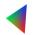The triangle area is 26.7 cm2. Determine the side length l if appropriate height hl = 45.3 cm.
3. Bathroom 2A bathroom is 2.4 meters long and 1.8 meters wide. How many square tiles 1 dm on each side are to be used to cover it?
4. RectangleThe rectangle area is 182 dm2, its base is 14 dm. How long is the other side? Calculate its perimeter.
5. 22/7 circleCalculate approximately area of a circle with radius 20 cm. When calculating π use 22/7.
6. Area of a triangleWhat is the area of a triangle that has the base 4 1/4 and the height of 3 3/3?
7. Area of RT 2Calculate the area of right triangle whose legs have a length 5.8 cm and 5.8 cm.
8. AnnulusThe radius of the larger circle is 8cm, the radius of smaller is 5cm. Calculate the contents of the annulus.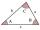The size of two internal angles of a triangle ABC are α=6/18π and β=7/18π. Calculate the size of the third angle.
10. Angles in triangle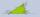Calculate the alpha angle in the triangle if beta is 61 degrees and 98 gamma degrees.
11. 8 degrees8 degrees Celsius it cools down by 9 degrees Celsius. What is the resultant temperature?
12. On SundayOn Sunday the temperature reached a high of 38° above 0. That night it dropped to 23° below 0. What is the difference between the high and low temperatures for Sunday?
13. The lowestThe lowest temperature on Thursday was 20°C. The lowest temperature on Saturday was 12°C. What was the difference between the lowest temperatures?
14. Bitoo and ReenaBitoo ate 3/5 part of an apple and the remaining part was eaten by his sister Reena. How much part of an apple did Renna eat? Who had the larger share? By how much?
15. Melting point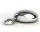The melting point of mercury is -36°F and its boiling point is 672°F. What is the difference between the boiling point and the melting point?
16. QuizQ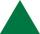An isosceles triangle has two sides of length 7 km and 39 km. How long is a third side?
17. 3-bracket 3Two angles in a triangle are 90° and 60°. Has triangle at least two equal sides?